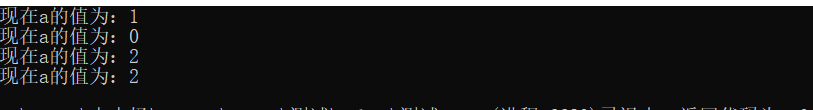# 第五次作业

## 2.作业过程

//
#include "pch.h"
#include <iostream>
using namespace std;
class clock {
public:
clock(int x = 0) :a(x) {}
void show()const { cout << "现在a的值为：" << a << endl; };
clock& operator++() {
a++;
return *this;
}
clock operator++(int) {
clock old = *this;
++(*this);
return old;
}
private:
int a;
};
int main()
{
clock x(0), y(0);
y = x++;
x.show();
y.show();
y = ++x;
x.show();
y.show();
return 0;
}## 3.作业总结

posted @ 2019-10-27 10:55  超级无敌57  阅读(80)  评论(0编辑  收藏  举报